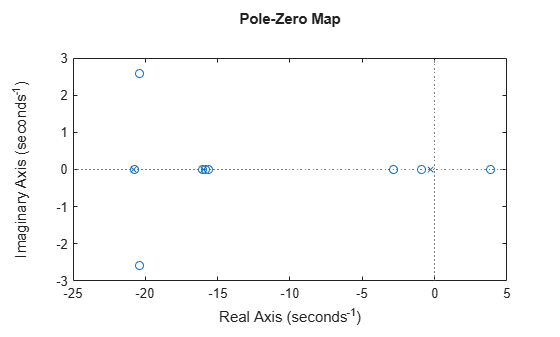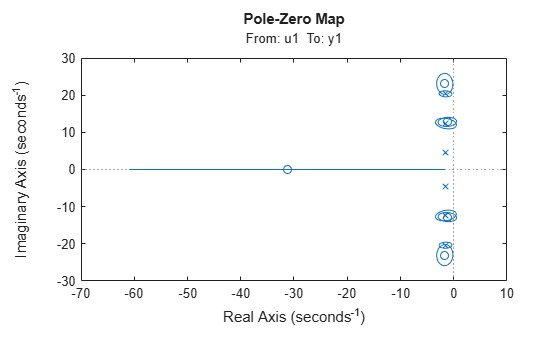# iopzplot

Plot pole-zero map for I/O pairs with additional plot customization options

## Syntax

``h = iopzplot(sys)``
``h = iopzplot(sys1,sys2,...,sysN)``
``h = iopzplot(sys1,LineSpec1,...,sysN,LineSpecN)``
``h = iopzplot(ax,...)``
``h = iopzplot(...,plotoptions)``

## Description

`iopzplot` lets you plot pole-zero maps for input/output pairs with a broader range of plot customization options than `iopzmap`. You can use `iopzplot` to obtain the plot handle and use it to customize the plot, such as modify the axes labels, limits and units. You can also use `iopzplot` to draw a pole-zero plot on an existing set of axes represented by an axes handle. To customize an existing plot using the plot handle:

1. Obtain the plot handle

2. Use `getoptions` to obtain the option set

3. Update the plot using `setoptions` to modify the required options

For more information, see Customizing Response Plots from the Command Line. To create pole-zero maps with default options or to extract pole-zero data, use `iopzmap`.

example

````h = iopzplot(sys)` plots the poles and zeros of each input/output pair of the dynamic system model `sys` and returns the plot handle `h` to the plot. `x` and `o` indicates poles and zeros respectively.```

example

````h = iopzplot(sys1,sys2,...,sysN)` displays the poles and transmission zeros of multiple models on a single plot. You can specify distinct colors for each model individually.```

example

````h = iopzplot(sys1,LineSpec1,...,sysN,LineSpecN)` sets the line style, marker type, and color for the plot of each system. All systems must have the same number of inputs and outputs to use this syntax.```
````h = iopzplot(ax,...)` plots into the axes specified by `ax` instead of the current axis `gca`.```

example

````h = iopzplot(...,plotoptions)` plots the poles and transmission zeros with the options specified in `plotoptions`. For more information on the ways to change properties of your plots, see Ways to Customize Plots.```

## Examples

collapse all

Create a pole/zero map of a two-input, two-output dynamic system.

```sys = rss(3,2,2); h = iopzplot(sys);```By default, the plot displays the poles and zeros of each I/O pair on its own axis. Use the plot handle to view all I/Os on a single axis.

`setoptions(h,'IOGrouping','all')`View the poles and zeros of a sixth-order state-space model estimated from input-output data. Use the plot handle to display the confidence intervals of the identified model's pole and zero locations.

```load iddata1 sys = ssest(z1,6,ssestOptions('focus','simulation')); h = iopzplot(sys); showConfidence(h)```There is at least one pair of complex-conjugate poles whose locations overlap with those of a complex zero, within the 1-σ confidence region. This suggests their redundancy. Hence, a lower (4th) order model might be more robust for the given data.

```sys2 = ssest(z1,4,ssestOptions('focus','simulation')); h = iopzplot(sys,sys2); showConfidence(h) legend('6th-order','4th-order') axis([-20, 10 -30 30])```The fourth-order model `sys2` shows less variability in the pole-zero locations.

## Input Arguments

collapse all

Dynamic system, specified as a SISO or MIMO dynamic system model, or an array of SISO or MIMO dynamic system models. Dynamic systems that you can use include continuous-time or discrete-time numeric LTI models such as `tf`, `zpk`, or `ss` models.

If `sys` is a generalized state-space model `genss` or an uncertain state-space model `uss`, `pzplot` returns the poles and transmission zeros of the current or nominal value of `sys`. If `sys` is an array of models, `pzplot` plots the poles and zeros of each model in the array on the same diagram.

Line style, marker, and color, specified as a character vector or string containing symbols. The symbols can appear in any order. You do not need to specify all three characteristics (line style, marker, and color). For example, if you omit the line style and specify the marker, then the plot shows only the marker and no line.

Example: `'--or'` is a red dashed line with circle markers

Line StyleDescription
`-`Solid line
`--`Dashed line
`:`Dotted line
`-.`Dash-dot line
MarkerDescription
`'o'`Circle
`'+'`Plus sign
`'*'`Asterisk
`'.'`Point
`'x'`Cross
`'_'`Horizontal line
`'|'`Vertical line
`'s'`Square
`'d'`Diamond
`'^'`Upward-pointing triangle
`'v'`Downward-pointing triangle
`'>'`Right-pointing triangle
`'<'`Left-pointing triangle
`'p'`Pentagram
`'h'`Hexagram
ColorDescription

`y`

yellow

`m`

magenta

`c`

cyan

`r`

red

`g`

green

`b`

blue

`w`

white

`k`

black

Axes handle, specified as an axes object. If you do not specify the axes object, then `pzplot` uses the current axes `gca` to plot the poles and zeros of the system.

Pole-zero plot options, specified as an options object. See `pzoptions` for a list of available plot options.

## Output Arguments

collapse all

Pole-zero plot options handle, returned as a scalar. Use `h` to query and modify properties of your pole-zero plot. You can use this handle to customize the plot with the `getoptions` and `setoptions` commands.

## Version History

Introduced before R2006a